Home | | Strength of Materials II | Strength of Materials- Advanced Topics In Bending of Beams

# Strength of Materials- Advanced Topics In Bending of Beams

Unsymmetrical bending of beams of symmetrical and unsymmetrical sections- curved beams- Winkler Bach formula- stress concentration- fatigue and fracture.

ADVANCED TOPICS IN BENDING OF BEAMS

1 UNSYMMETRICAL BENDING

The plane of loading (or) that of bending does not lie in (or) a plane that contains  the principle centroidal axis of the cross- section; the bending is called Unsymmetrical bending.

2 STATE THE TWO REASONS FOR UNSYMMETRICAL BENDING

(i) The section is symmetrical (viz. Rectangular, circular, I section) but the load  line is inclined to both the principal axes.

(ii) The section is unsymmetrical (viz. Angle section (or) channel section vertical  web) and the load line is along any centroidal axes.

3.SHEAR CENTRE

The shear centre (for any transverse section of the beam) is the point of  intersection of the bending axis and the plane of the transverse section. Shear centre is  also known as 'centre of twist'

4.WRITE THE SHEAR CENTRE EQUATION FOR CHANNEL SECTIONe = Distance of the shear centre (SC ) from the web along the symmetric axis XX

Aw = Area of the web

Af = Area of the flange

A CHANNEL SECTION HAS FLANGES 12 CM X 2 CM AND WEB 16 CM X 1  CM. DETERMINE THE SHEAR CENTRE OF THE CHANNEL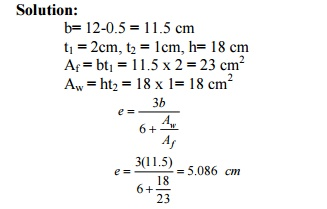5 WRITE THE SHEAR CENTRE EQUATION FOR UNSYMMETRICAL I SECTIONe = Distance of the shear centre (SC) from the web along the symmetric axis XX  t1 = thickness of the flange

h = height of the web

b1 = width of the flange in right portion.

b2 = width of the flange in left portion.

Ixx = M.O.I of the section about XX axis.

6 DERIVE THE EQUATION OF SHEAR CENTRE FOR CHANNEL SECTION

A channel section (flanges: b x t1  ; Web h x t2)  with XX as the horizontal symmetric axis.

Let S = Applied shear force. (Vertical downward X)

(Then S is the shear force in the web in the upward direction)

S1 = Shear force in the top flange (there will be equal and opposite shear force  in the bottom flange as shown.)

Now, shear stress (t) in the flange at a distance of x from the right hand edge (of the top flange)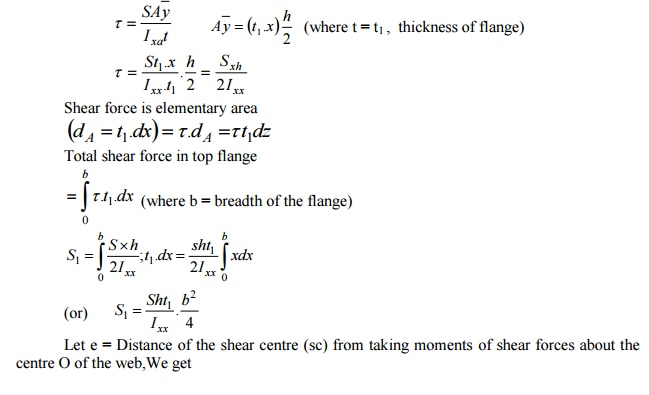7 DERIVE THE EQUATION OF SHEAR CENTER FOR UNEQUAL-SECTION Solution:

An unequal I -section which is symmetrical about XX axis.

Shear stress in any layer,8 DERIVE THE STRESSES IN CURVED BARS US ING WINKLER-BACH THEORY

The simple bending formula, however, is not applicable for deeply curved beams where  the neutral and centroidal axes do not coincide. To deal with such cases Winkler -Bach Theory  is used.

A bar ABCD initially; in its unstrained   state.  Let   AB?CD?   be   th bar.

Let R = Radius of curvature of the centroidal axis HG.

Y       =       Distance of the fiber EF from the centroidal layer HG.

R?   =  Radius   of   curvature   of   HG?

M      =       Uniform bending moment applied to the beam (assumed  positive when tending to increase the curvature)

R?   =         Original angle subtended by the centroidal axis HG at its  centre of curvature O and

q? =  Angle   subtended   by   HG?   (after   ben  q?

For finding the strain and stress normal to the section, consider the fibre EF at a distance  y from the centroidal axis.

Let ? be the stress in the strained layer EF  same layer.The curved member shown in fig. has a solid circular cross -section 0.01 m in diameter.  If the maximum tensile and compressive stresses in the member are not to exceed 150  MPa and 200 MPa. Determine the value of load P that can safely be carried by the member.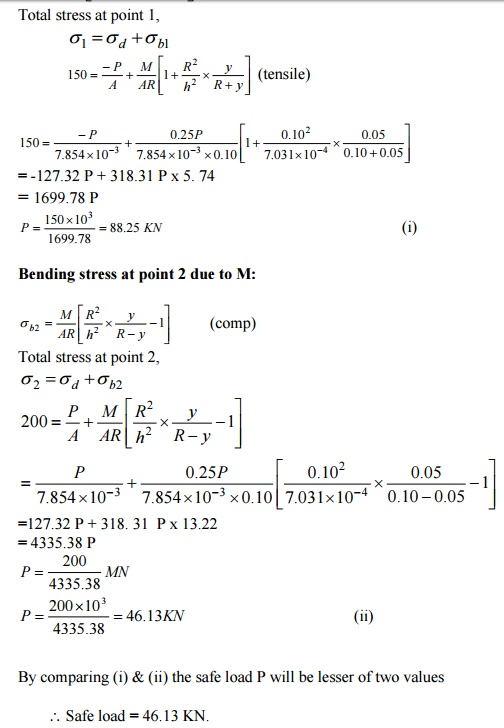A frame subjected to a load of 2.4 kN. Find (i) The resultant stresses at a point 1 and  2;   (ii) Position of neutral axis.A curved bar is formed of a tube of 120 mm outside diameter and 7.5 mm thickness. The  centre line of this is a circular arc of radius 225 mm. The bending moment of 3 kNm  tending to increase curvature of the bar is applied. Calculate the maximum tensile and  compressive stresses set up in the bar.A CURVED BEAM HAS A T-SECTION. THE INNER RADIUS IS 300 MM. WHAT IS  THE ECCENTRICITY OF THE SECTION?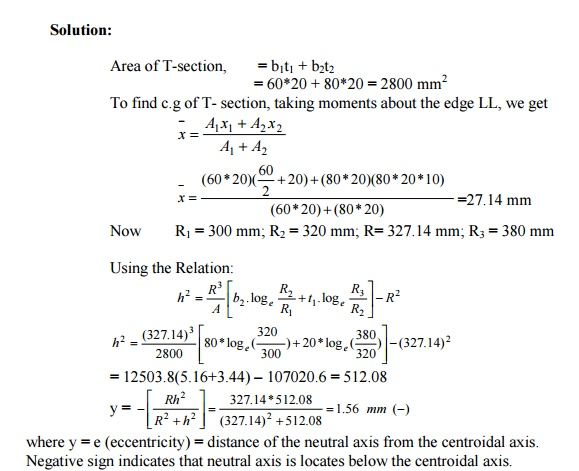C- FRAME SUBJECTED TO A LOAD OF 120 KN. DETERMINE THE STRESSES AT A  AND B.DERIVE THE FORMULA FOR THE DEFLECTION OF BEAMS DUE TO

UNSYMMETRICAL BENDING

Solution:

Tthe transverse section of the beam with centroid G. XX and YY are two  rectangular co-ordinate   axes   and   UU   and   VV   are   the   pri  XY set of co-ordinates axes. W is the load acting along the line YY on the section of the beam.  The load W can be resolved into the following two components:

(i)                         W   sin   ?   ��   along   UG

(ii)                       W   cos   ?   ��   along   VG

Let,u=  Deflection?   caused   by   the   component   W   si

VV axis, and

v =   Deflection   caused  long by the thelineGV due component to bendingabodt   W   co

UU axis.

Then  depending   upon   the   end  u conditionsandvare?givenby   of   thwhere, K = A constant depending on the end conditions of the  beam and position of the load along the beam, and

l = length of the beam

The    total   or   resultant   deflection   ?   can   thenA 80 mm x 80 mm x 10 mm angle section shown in fig. is used as a simply supported beam  over a span of 2.4 m. It carries a load of 400 kN along the line YG, where G is the centroid  of the section. Calculate (i) Stresses at the points A, B and C of the mid -section of the  beam (ii) Deflection of the beam at the mid-section and its direction with the load line (iii)  Position of the neutral axis. Take E = 200 GN/m2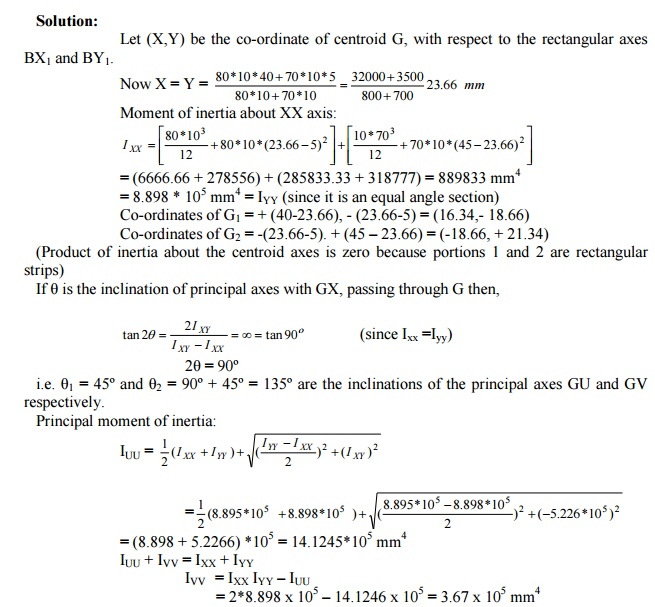10 STATE THE PARALLEL AXES AND PRINCIPAL MOMENT OF INERTIA

If the two axes about which the product of inertia is found, are such , that the  product of inertia becomes zero, the two axes are then called the principle axes. The  moment of inertia about a principal axes is called the principal moment of inertia.

11 STRESS CONCENTRATION

The term stress gradient is used to indicate the rate of increase of stress as a stress  raiser is approached. These localized stresses are called stress concentration.

12 STRESS- CONCENTRATION FACTOR

It is defined as the ratio of the maximum stress to the nominal stress.?max = maximum stress

?nom = nominal stress

13 FATIGUE STRESS CONCENTRATION FACTOR

The fatigue stress -concentration factor (Kf ) is defined as the ratio of flange limit  of unnotched specimen to the fatigue limit of notched specimen under axial (or) bending loads.

K f  =1+q(Kt  -1)

Value of q ranges from zero to one.

14 SHEAR FLOW

Shear flow is defined as the ratio of horizontal shear force H over length of the  beam x. Shear flow is acting along the longitudinal surface located at discharge y1.Shear  flow is defined by q.H = horizontal shear force

15 EXPLAIN THE POSITION OF SHEAR CENTRE IN VARIOUS SECTIONS

(i) In case of a beam having two axes of symmetry, the shear centre coincides with the centroid.

(ii)  In case of sections having one axis of symmetry, the shear centre does not  coincide with the centroid but lies on the axis of symmetry.

16 STATE THE PRINCIPLES INVOLVED IN LOCATING THE SHEAR CENTRE

The principle involved in locating the shear centre for a cross -section of a beam is that the loads acting on the beam must lie in a plane which contains the resultant shear  force on each cross-section of the beam as computed from the shearing stresses.

DETERMINE THE POSITION OF SHEAR CENTRE OF THE SECTION OF  THE BEAM

Solution:

t1 = 4 cm, b1 = 6 cm, b2 = 8 cm  h1 = 30 -4 = 26 cm17 STATE THE STRESSES DUE TO UNSYMMETRICAL BENDING?b = bending stress in the curved bar

M = moment due to the load applied

IUU = Principal moment of inertia in the principal axes UU

I VV = Principal moment of inertia in the principal axes VV

18 FATIGUE

19 TYPES OF FATIGUE STRESS

(i)  Direct stress

(ii)  Plane bending

(iii)  Rotating bending

(iv) Torsion

(v)  Combined stresses

(a) Fluctuating or alternating stress

(b)  Reversed stress.

20 STATE THE REASONS FOR STRESS- CONCENTRATION

When a large stress gradient occurs in a small, localized area of a structure, the  high stress is referred to as a stress concentration. The reasons for stress concentration are

(i) discontinuities in continuum

(ii) contact forces.

21 CREEP

Creep can be defined as the slow and progressive deformation of a material with  time under a constant stress.

Study Material, Lecturing Notes, Assignment, Reference, Wiki description explanation, brief detail
Civil : Strength of Materials : Advanced Topics In Bending of Beams : Strength of Materials- Advanced Topics In Bending of Beams |